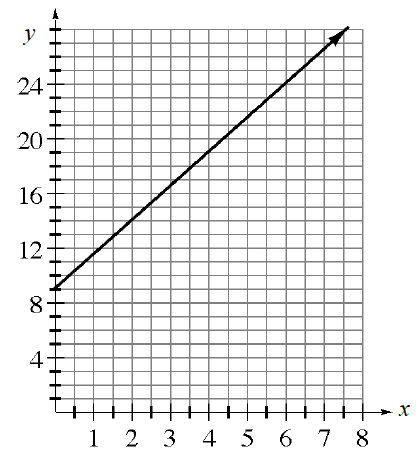### Home > CCAA8 > Chapter 5 Unit 6 > Lesson CCA: 5.3.1 > Problem5-90

5-90.This problem is a checkpoint for writing the equation of a line. It will be referred to as Checkpoint 5B.

Use the given information to find an equation of the line.

1. Slope 2 and passing through (10, 17).

2. Passing through (1, –4) and (–2, 5).

3.  $x$ $y$ $–6$ $–3$ $0$ $3$ $6$ $–6$ $–4$ $–2$ $0$ $2$
4.Check your answers by referring to the Checkpoint 5B materials located at the back of your book.

Ideally, at this point you are comfortable working with these types of problems and can solve them correctly. If you feel that you need more confidence when solving these types of problems, then review the Checkpoint 5 materials and try the practice problems provided. From this point on, you will be expected to do problems like these correctly and with confidence.

Answers and extra practice are located in the back of your printed textbook or in the Reference Tab of your eBook.
If you have an eBook for CCA, login and then click the following link: